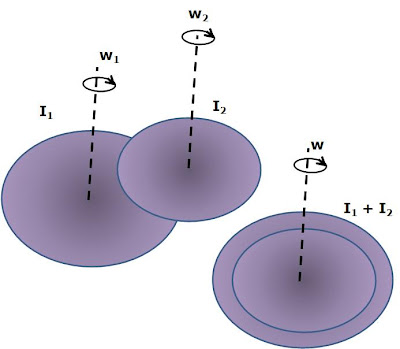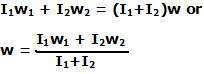## Sunday, February 15, 2009

### Irodov Problem 1.278As soon as the discs are put on each other, friction forces start to act between them to eliminate their relative motion. These friction forces generate equal and opposite torques on the two discs. This will decelerate one of the discs and accelerate the other until both spin at the same angular velocity and there is not relative motion between them.

Since friction forces are completely internal to the two disc system, there is no external torque acting on the two disc system. Consequently, the total angular momentum of the two disc system must be conserved at all times. Initially, the total angular momentum of the two disc system is I1w1 + I2w2. The moment of inertia of the two disc system when they are spinning together is I1+I2. Let the final angular velocity be w. Hence, we have,The work done by friction is entirely responsible for reducing the total kinetic energy of the two disc system and is equal to the final kinetic energy - initial kinetic energy given by,